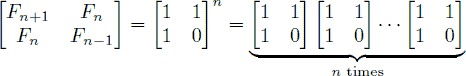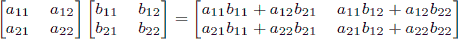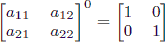Online JudgeProblem SetAuthorsOnline ContestsUser
Web Board
F.A.Qs
Statistical Charts
Problems
Submit Problem
Online Status
Prob.ID:
Register
Authors ranklist
Current Contest
Past Contests
Scheduled Contests
Award Contest
Register
Language:
Fibonacci
 Time Limit: 1000MS Memory Limit: 65536K Total Submissions: 29740 Accepted: 19608

Description

In the Fibonacci integer sequence, F0 = 0, F1 = 1, and Fn = Fn − 1 + Fn − 2 for n ≥ 2. For example, the first ten terms of the Fibonacci sequence are:

0, 1, 1, 2, 3, 5, 8, 13, 21, 34, …

An alternative formula for the Fibonacci sequence is.

Given an integer n, your goal is to compute the last 4 digits of Fn.

Input

The input test file will contain multiple test cases. Each test case consists of a single line containing n (where 0 ≤ n ≤ 1,000,000,000). The end-of-file is denoted by a single line containing the number −1.

Output

For each test case, print the last four digits of Fn. If the last four digits of Fn are all zeros, print ‘0’; otherwise, omit any leading zeros (i.e., print Fn mod 10000).

Sample Input

```0
9
999999999
1000000000
-1```

Sample Output

```0
34
626
6875```

Hint

As a reminder, matrix multiplication is associative, and the product of two 2 × 2 matrices is given by.

Also, note that raising any 2 × 2 matrix to the 0th power gives the identity matrix:.

Source

[Submit]   [Go Back]   [Status]   [Discuss]Home PageGo BackTo top